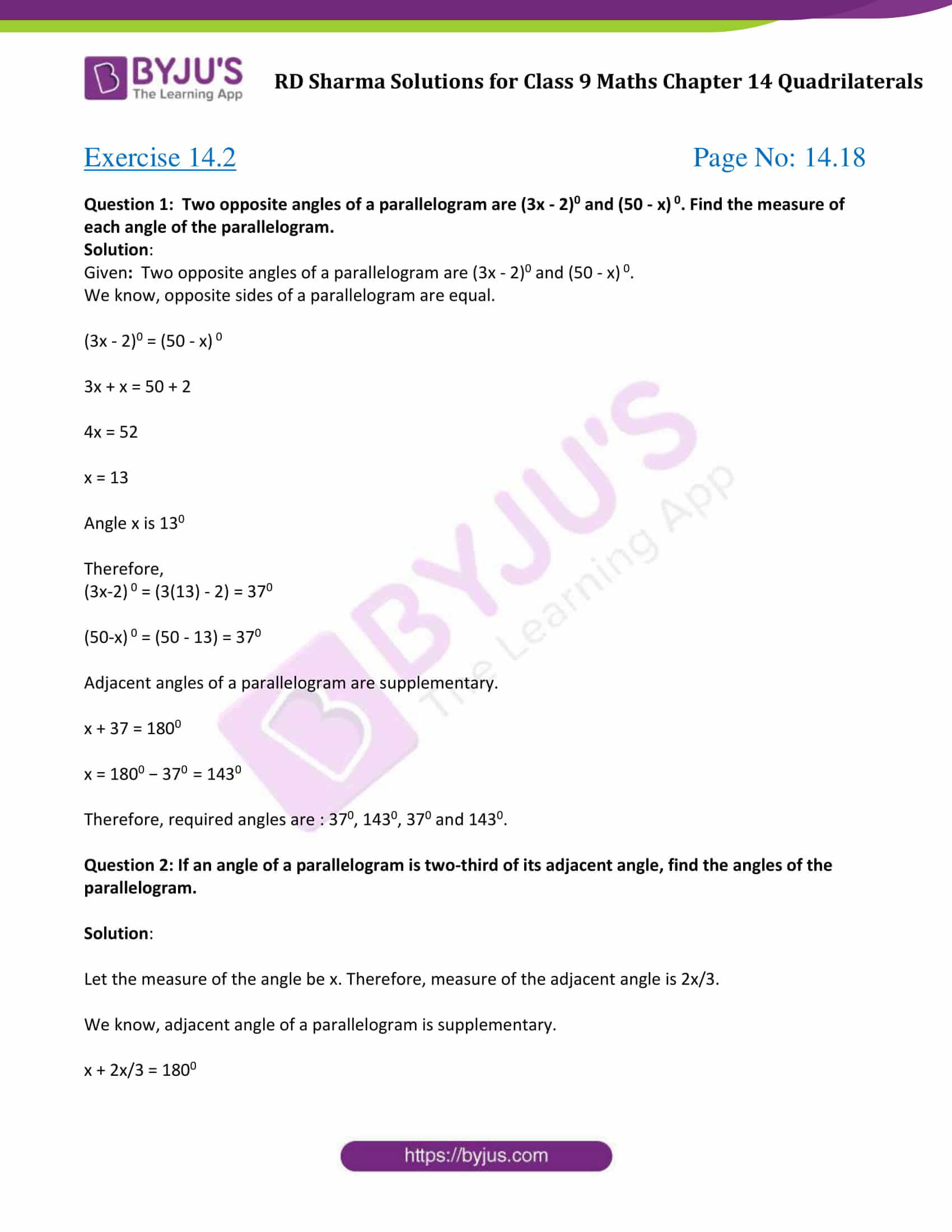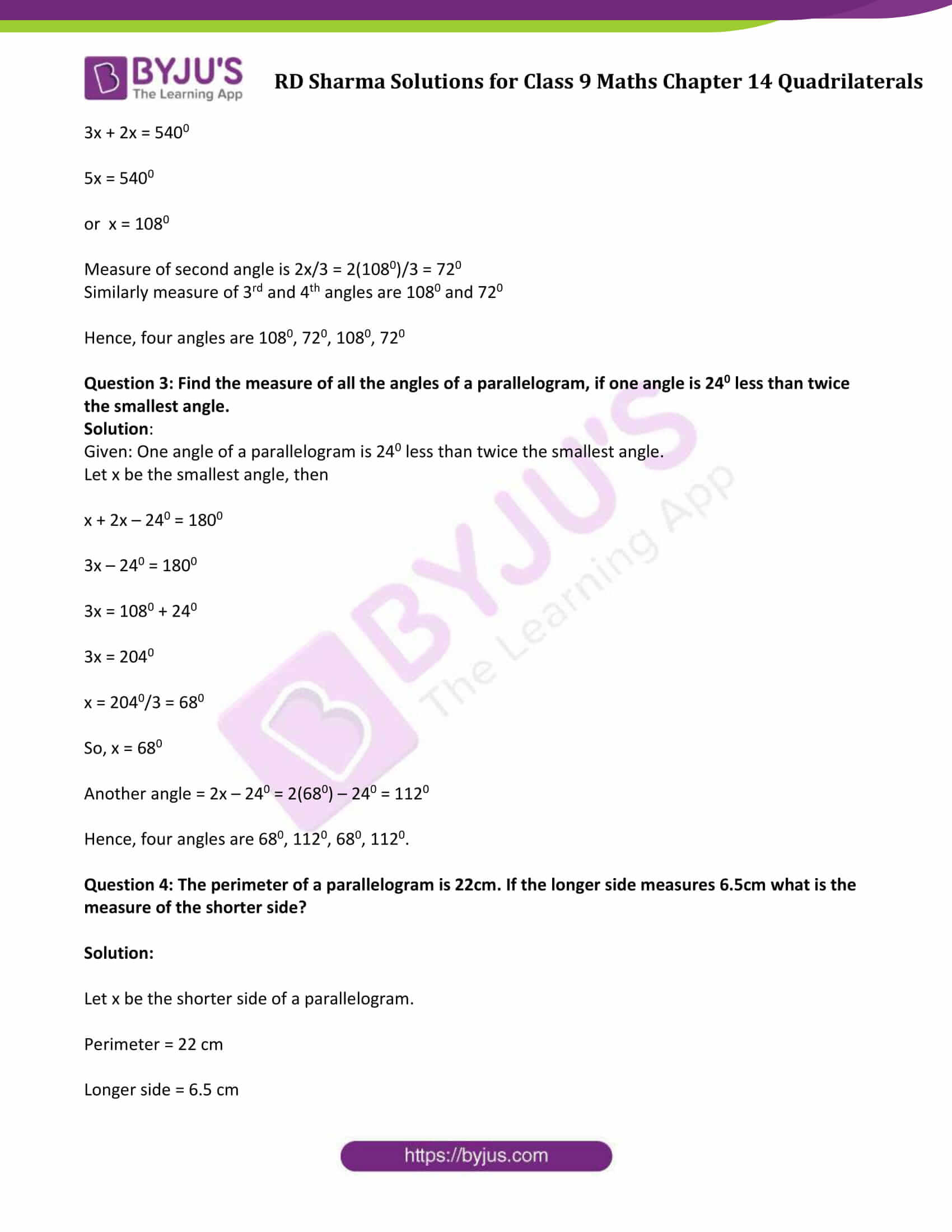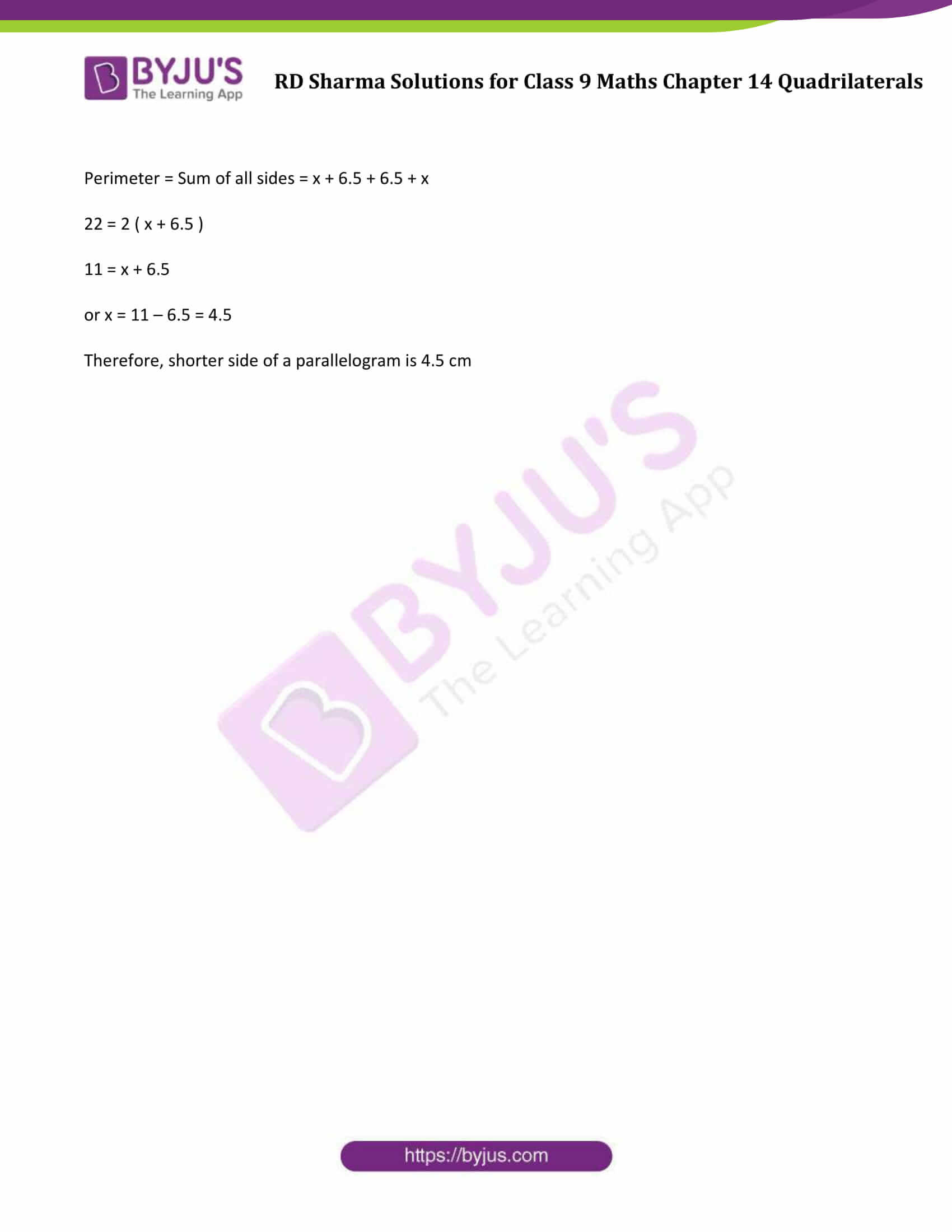# RD Sharma Solutions for Class 9 Maths Chapter 14 Quadrilaterals Exercise 14.2

RD Sharma Class 9 Mathematics Chapter 14 Exercise 14.2 Solutions for Quadrilaterals are provided here. In this exercise, students will learn about various types of quadrilaterals like rectangle, square, rhombus and many more. RD Sharma solutions given here include the solutions of all the questions enlisted under exercise 14.2. Students are advised to click on the below link and download pdf now.### Access Answers to Maths RD Sharma Solutions for Class 9 Chapter 14 Quadrilaterals Exercise 14.2 Page number 14.18

Question 1: Two opposite angles of a parallelogram are (3x – 2)0 and (50 – x) 0. Find the measure of each angle of the parallelogram.

Solution:

Given: Two opposite angles of a parallelogram are (3x – 2)0 and (50 – x) 0.

We know, opposite sides of a parallelogram are equal.

(3x – 2)0 = (50 – x) 0

3x + x = 50 + 2

4x = 52

x = 13

Angle x is 130

Therefore,

(3x-2) 0 = (3(13) – 2) = 370

(50-x) 0 = (50 – 13) = 370

Adjacent angles of a parallelogram are supplementary.

x + 37 = 1800

x = 1800 − 370 = 1430

Therefore, required angles are : 370, 1430, 370 and 1430.

Question 2: If an angle of a parallelogram is two-third of its adjacent angle, find the angles of the parallelogram.

Solution:

Let the measure of the angle be x. Therefore, measure of the adjacent angle is 2x/3.

We know, adjacent angle of a parallelogram is supplementary.

x + 2x/3 = 1800

3x + 2x = 5400

5x = 5400

or x = 1080

Measure of second angle is 2x/3 = 2(1080)/3 = 720

Similarly measure of 3rd and 4th angles are 1080 and 720

Hence, four angles are 1080, 720, 1080, 720

Question 3: Find the measure of all the angles of a parallelogram, if one angle is 240 less than twice the smallest angle.

Solution:

Given: One angle of a parallelogram is 240 less than twice the smallest angle.

Let x be the smallest angle, then

x + 2x – 240 = 1800

3x – 240 = 1800

3x = 1080 + 240

3x = 2040

x = 2040/3 = 680

So, x = 680

Another angle = 2x – 240 = 2(680) – 240 = 1120

Hence, four angles are 680, 1120, 680, 1120.

Question 4: The perimeter of a parallelogram is 22cm. If the longer side measures 6.5cm what is the measure of the shorter side?

Solution:

Let x be the shorter side of a parallelogram.

Perimeter = 22 cm

Longer side = 6.5 cm

Perimeter = Sum of all sides = x + 6.5 + 6.5 + x

22 = 2 ( x + 6.5 )

11 = x + 6.5

or x = 11 – 6.5 = 4.5

Therefore, shorter side of a parallelogram is 4.5 cm

## RD Sharma Solutions for Class 9 Maths Chapter 14 Quadrilaterals Exercise 14.2

RD Sharma Solutions Class 9 Maths Chapter 14 Quadrilaterals Exercise 14.2 is based on the following topics and subtopics:

– Trapezium

– Isosceles Trapezium

– Parallelogram

– Rhombus

– Rectangle

– Square

• Properties of Parallelogram
• Some important theorems on Quadrilaterals